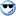View Single Post
 teshRegistered User Join Date: Feb 2007 Location: , , . Posts: 1 Thanks: 0 Thanked 0 Times in 0 PostsBug or no to bug learning C using VS.Net to compil

Gurus.

I have written the following code with the help of Ivor Horton's Beginning C :

// Structures, Arrays of Structures.

#include "stdafx.h"
#include "stdio.h"
#define MY_ARRAY 15
#define BASIS 360

struct swap
{
char counterParty;
float notional;
float intRate;
int busDays;
//int basis = 360;
float flows;
};

void main()
{

/*Arrays of Structures*/

swap myArraySwap[MY_ARRAY];

int hcount = 0;
int i = 0;
//='\0';

// for (hcount = 0; hcount < 5 ; hcount++ )
do
{
char test = NULL;
printf("\nWould you like to enter Swaps (Y or N)?:");

test = getchar();

if (test != 'Y') //|| test !='y')
break;

printf("\nEnter CounterParty:");
scanf("%s", &myArraySwap[hcount].counterParty );
printf("\nEnter Notional :" );
scanf("%f", &myArraySwap[hcount].notional );
printf("\nEnter Interest Rate:" );
scanf("%f", &myArraySwap[hcount].intRate );
printf("\nEnter Number of days:" );
scanf("%d", &myArraySwap[hcount].busDays );
myArraySwap[hcount].flows = (myArraySwap[hcount].notional * (myArraySwap[hcount].intRate/100.0f) * ((float)myArraySwap[hcount].busDays/360.0f));

hcount++;
}
while (hcount < 5);

for (i=0 ; i < hcount ; i++)
{
printf("\nCounter Party: %s",myArraySwap[hcount].counterParty);
printf("\nFlows %f", myArraySwap[i].flows);
printf("\nDone");
}

}

Problem:

First time around the var test = '' and I can enter a value i.e. Y

here is the dos output:

Would you like to enter Swaps (Y or N)?:Y

Enter CounterParty:Test

Enter Notional :1000

Enter Interest Rate:5.25

Enter Number of days:35

Would you like to enter Swaps (Y or N)?:

second time around the at the point of test = getchar(); test is set to '0 ' automatically and I am not able to enter Y or N.

when the app reaches:test = getchar(); it automatically sets the var from Y 89 to : 0 ''

is this compiler related i have vs.net 2003 ?

i have also tried scanf and get the same :(

thank you.

tesh